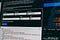# Web Scraping From Scratch With 3 Simple StepsPhoto by Pankaj Patel on Unsplash

## Understand the structure of your target website

`<section class="cex-table"> <section class="thead">  <div>...</div>  <div class="tr-wrapper">   <div class="tr-left">    <div class="tr">     <div>...</div>     <div style="flex:7" class="th">      <span class="cell">      <i class="sorting-icon">      </i>      <span class="cell-text">Asset</span>      </span>     </div>    </div>   </div>  </div>  ... </section></section>`
`<section class="tbody"> <section class="tr-section">  <a href="/price/bitcoin">   <div class="tr-wrapper">    <div class="tr-left">     <div class="tr">      <div style="flex:2" class="td">       <span class="cell cell-rank">       <strong>01</strong>       </span>      </div>      <div style="flex:7" class="td">       <span class="cell cell-asset">       <img>...</img>       <strong class="cell-asset-title">Bitcoin</strong>       <span class="cell-asset-iso">BTC</span>       </span>      </div>     </div>    </div>   </div>  </a> </section></section>`

## Locate and parse the target data element with XPath

`pip install requests pip install lxml`
`import requests from lxml import html target_url = "https://www.coindesk.com/coindesk20" result = requests.get(target_url)`
`payload = {"q" : "bitcoin", "s" : "relevant"} result = requests.get("https://www.coindesk.com/search", params=payload)`
`tree = html.fromstring(result.text)`
`table_header = tree.xpath("//section[@class='cex-table']/section[@class='thead']/div[@class='tr-wrapper']")`
`headers = table_header.xpath(".//span[@class='cell']/span[@class='cell-text']/text()")`
`['Asset', 'Price', 'Market Cap', 'Total Exchange Volume', 'Returns (24h)', 'Total Supply', 'Category', 'Value Proposition', 'Consensus Mechanism']`
`table_body = tree.xpath("//section[@class='cex-table']/section[@class='tbody']/section[@class='tr-section']")`
`records = [] for row in table_body:     tokens = row.xpath(".//span[contains(@class, 'cell-asset-iso')]/text()")    ranks = row.xpath(".//span[contains(@class, 'cell-rank')]/strong/text()")    assets = row.xpath(".//span[contains(@class, 'cell-asset')]/strong/text()")    spans = row.xpath(".//div[contains(@class,'tr-right-wrapper')]/div/span[contains(@class, 'cell')]")    rest_cols = [span.text_content().strip() for span in spans]    row_data = ranks + tokens + assets + rest_cols   records.append(row_data)`
`column_header = ["Rank", "Token"] + headers`

## Save the scraping result

`import pandas as pd df = pd.DataFrame(records, columns=column_header)`
`import csv with open("token_price.csv", "w", newline="") as csvfile:     writer = csv.writer(csvfile)     writer.writerow(column_header)     for row in records:         writer.writerow(row)`

## Conclusion

Resources and tutorials for python, data science and automation solutions

## More from codeforests

Resources and tutorials for python, data science and automation solutions